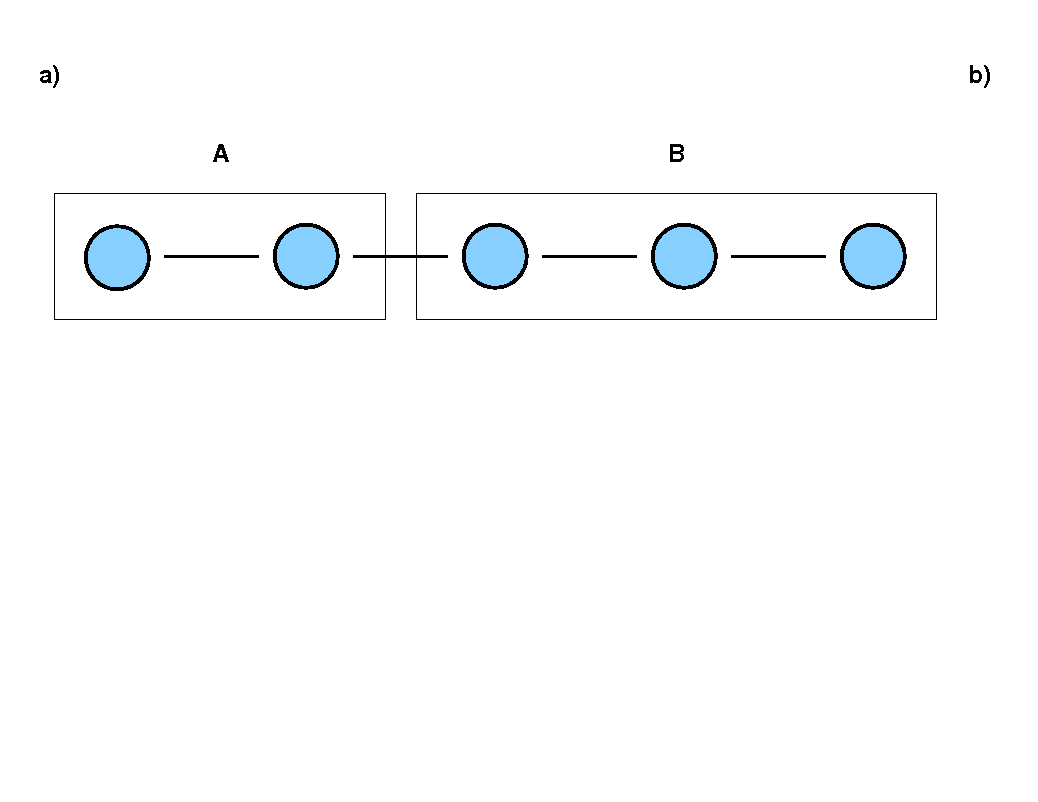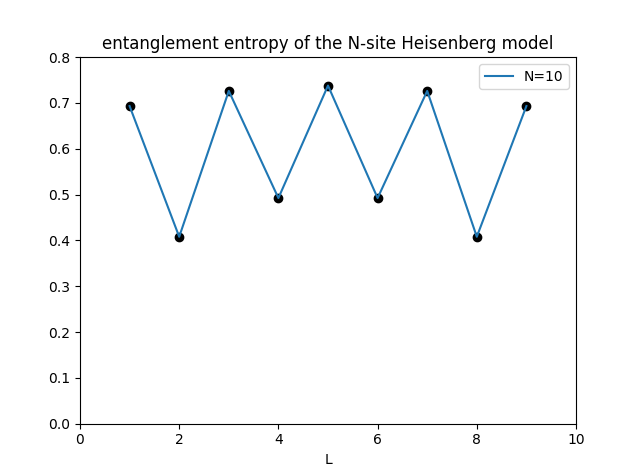# Thesis projects

Here is a list of topics suitable for bachelor and master thesis projects:

## Entanglement entropy in the random Heisenberg model

Basically all eigenstates of many-body Hamiltonians show some degree of entanglement - in other words, product states are rare exceptions. Now consider the one-dimensional Heisenberg model and a division of the system in two parts (A and B), as shown in Fig. a). The entanglement entropy Se measures the degree of entanglement between the subsystems A and B and its L-dependence (L is the number of sites in A) is shown in Fig. b) for the groundstate of the antiferromagnetic case. The question to be studied in this project is how the entanglement properties change in a random Heisenberg model, with the randomness introduced, for example, by setting the nearest-neighbour couplings Jn as random numbers in some interval Jmin < Jn < Jmax. Averaging over many sets of {Jn} gives a distribution P(Se).## Iterative diagonalization of the Heisenberg chain

The Schrödinger equation for a cluster of interacting quantum particles can be solved by diagonalization of the corresponding Hamilton matrix, provided the numerical diagonalization of this matrix does not exceed the limits of computing time and memory. In this exact (or full) diagonalization approach, a single matrix is set up for the whole system. In the iterative diagonalization approach, however, one site of the system is added in each iteration - the enlarged cluster is then diagonalized using the information that has been kept from the previous iteration. Combined with a suitable truncation scheme, the iterative diagonalization allows to treat much larger system sizes. The aim of this project is to set up such an iterative diagonalization for the one-dimensional Heisenberg model. Various truncation schemes can be used here, based on the spectrum of eigenenergies or the entanglement spectrum.

## Zero-point entropy of quantum impurity systems

The thermodynamic entropy of a quantum system in the limit of temperature to zero is given by S(T to 0) = ln(dg), with dg the degeneracy of the ground state. This degeneracy can only have integer values, dg=1,2,3,... There are, however, quantum impurity systems for which dg seems to acquire non-integer values: as an example, the two-channel Kondo model has a zero-point entropy of Simp = 0.5 ln(2), corresponding to dg=sqrt(2). The idea of this project is to derive this anomalous value of Simp from the specific fixed point structure of the two-channel Kondo model.

## Entanglement in fermionic systems

A model of non-interacting fermions, such as the one-dimensional tight-binding model, can be easily transformed between a real-space representation (with the fermionic operators acting on individual sites) and a representation in k-space. This transformation can be used to express quantum impurity models (here: the single-impurity Anderson model) in different representations: the site- and k-representation. To investigate the entanglement properties of a quantum impurity model (e.g.: "How strongly is subsystem A entangled with subsystem B"), the representation of the fermionic part has to be specified and the results will in fact depend on this choice. The focus of this project is the single-impurity Anderson model on a small cluster such that the Hamilton matrix of the model can be diagonalized exactly. For this model, the entanglement entropy should be calculated for different representations and different divisions in subsystems A and B. The results will be useful to decide which representation is best suited to characterize the entanglement structure of quantum impurity systems. (See also the paper by Chun Yang and Adrian E. Feiguin, Unveiling the internal entanglement structure of the Kondo singlet, Phys. Rev. B 95, 115106 (2017).)